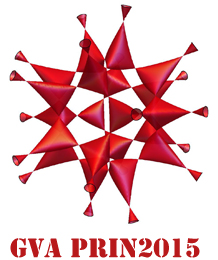Nodes

 Node Principal investgator Research themes Catania Francesco Russo 1) A- Classical methods in algebraic geometry. 2) A- Lefschetz properties of Artin algebras. 3) A- Artin algebras of 0-dimensional schemes in Segre products. 4) C- Geography of surfaces of general type with isotrivial fibrations. Ferrara Massimiliano Mella 1) A- Projective and birational classification. 2) A- Birational geometry of uniruled varieties. 3) C- Families of curves on algebraic surfaces. 4) F- Tensor decomposition and identifiability. Firenze Massimiliano Mella 1) A- Classical methods in algebraic geometry. 2) A- Hilbert schemes of points in Segre products. 3) F- Tensor decompositions with applications. 4) F- Effective numerical methods in algebraic geometry. Genova Aldo Conca 1) A- Syzygies theory and methods of commutative algebra. 2) A- Positivity conditions in adjunction theory. 3) D- Classification of elliptic Calabi Yau fibrations. 4) F- Methods of computational algebraic geometry. Milano Bert Van Geemen 1) A- Adjunction theory and birational classification. 2) B- Moduli spaces of curves and related topics. 3) C- Geometry and moduli of hyperkahler varieties. 4) D- Calabi Yau geometry, with derived categories. Pisa Rita Pardini 1) A- Syzygies theory and methods of commutative algebra. 2) B- Curves on algebraic surfaces and their moduli. 3) C- Geography and moduli of surfaces of general type. Roma Tre Alessandro Verra 1) A- Rationality problems for varieties and moduli. 2) A- Birational geometry in higher dimensions. 3) B- Curves and moduli spaces related to curves. 4) E- Tropicalization of moduli spaces. RomaTor Vergata Flaminio Flamini 1) A- Classical projective methods in algebraic geometry. 2) A- Cremona transformations. 3) B- Families of curves on K3 surfaces. 4) D- Compactifications of families of hyperkahler varieties. S.I.S.S.A Ugo Bruzzo 1) B- Moduli of linear series on curves: compactifications and wall crossing. 2) D- Gromov-Witten invariants of weighted c.i. Calabi Yau 3-folds. 3) D- Moduli of framed sheaves and interactions with physics. Politecnico di Torino Gianfranco Casnati 1) A- Birational geometry of Fano varieties. 2) A- Hilbert schemes of points in Segre products. 3) A- Effective parametrizations of Hilbert schemes. 4) F- Tensor decomposition with applications to several fields. Trento Marco Andreratta 1) A- Birational and projective methods of classification. 2) B- Moduli of curves and related topics. 3) C- Geography of surfaces, and threefolds, of general type. 4) F- Combinatorial methods in algebraic geometry with applications. Trieste Emilia Mezzetti 1) A- Pseudo-automorphims of rational varieties and group actions. 2) A- Birational and projective classification of algebraic varieties. 3) A- Lefschetz properties of Artinian algebras. 4) D- Effective methods in algebraic geometry.
A M.I.U.R   PRIN 2015 project.
Webmaster: Valerio Talamanca
Send comments to: valerio at mat.uniroma3.it